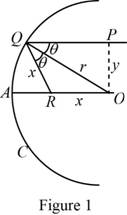# The change in point R as P is taken closer and closer to the x -axis.### Single Variable Calculus: Concepts...

4th Edition
James Stewart
Publisher: Cengage Learning
ISBN: 9781337687805### Single Variable Calculus: Concepts...

4th Edition
James Stewart
Publisher: Cengage Learning
ISBN: 9781337687805

#### Solutions

Chapter 3, Problem 19P
To determine

## The change in point R as P is taken closer and closer to the x-axis.

Expert Solution

The point R approaches the midpoint of radius AO when P is taken closer and closer to the x-axis.

### Explanation of Solution

Draw the vertical line from P to O in the given graph as shown below in Figure 1.From Figure 1, the angle PQO=OQR=θ.

The triangle QOR is isosceles.

Thus, |QR|=|RO|.

Let |QR|=x and |QO|=r.

The cosine law of the triangle QOR is,

|RO|2=|QR|2+|QO|22|QR||QO|cosθ

Since |QR|=|RO|,

x2=x2+r22xrcosθ2xrcosθ=r2x=r22rcosθx=r2cosθ

From Figure 1, assume that the line |PO|=y.

sinθ=yr

The point P is closer and closer to the x-axis, that is, |PO|=y is approaching to 0, then sinθ=yr approach zero and the value θ also approaches to zero.

If θ approaches to zero, then x=r2cosθ approaches to r2.

Since AO is a radius of the circle, |AO|=r.

If the point P is closer and closer to the x-axis, then x approach r2.

That is, R approach AO2.

Therefore, the point R approaches the midpoint of radius AO.

### Have a homework question?

Subscribe to bartleby learn! Ask subject matter experts 30 homework questions each month. Plus, you’ll have access to millions of step-by-step textbook answers!